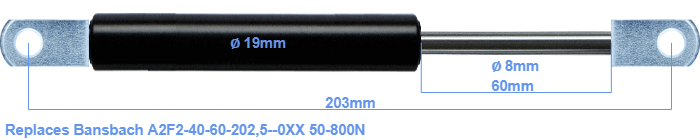# Replacement for Bansbach A2F2-40-60-202,5–0XX 50-800N

\$28.16

Replacement gas spring for the Bansbach A2F2-40-60-202,5--0XX 50-800 Newton. The eye has a thickness of 10mm and a hole diameter of 8.1mm. Brand: Stabilus Industry Line.

Please note:
Mounting part rod : The thickness of the eye of this replacement is 10mm instead of 18mm.
 Force Choose an option50 Newton60 Newton80 Newton100 Newton120 Newton140 Newton150 Newton160 Newton180 Newton200 Newton220 Newton240 Newton250 Newton260 Newton280 Newton300 Newton320 Newton340 Newton350 Newton360 Newton380 Newton400 Newton420 Newton440 Newton450 Newton460 Newton480 Newton500 Newton520 Newton540 Newton550 Newton560 Newton580 Newton600 Newton620 Newton640 Newton650 Newton660 Newton680 Newton700 Newton720 Newton740 Newton750 Newton760 Newton780 Newton800 NewtonClearThe bore of the house of this replacement gas spring is 19 mm. The cross section of the rod is 8 mm. The stroke is 60 millimeter long. The total length of this replacement gas spring is 203 mm. Note: this is the total length between the rotation points of the two mounting parts. Without the mounting parts this gas spring is 165 mm long (thread to thread). The force of this replacement is 50-800 Newton. This is not an original gas spring from Bansbach, but a so called replacement gas spring. This replacement gas spring is a Stabilus Industry Line gas spring. Nevertheless, this gas spring can be used as replacement gas spring, because the dimensions and forces are close to equal.